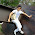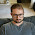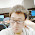## Linked List Cycle II

Given a linked list, return the node where the cycle begins. If there is no cycle, return null.
Can you solve it without using extra space?

### Analysis:

This problem can be viewed as two major steps:
(1) Detect whether the loop exists in the linked list.
(2) Find the loop start node if loop exists.

The (1) step can be easily solved using the slow&fast pointers (see Linked List Cycle)
How to deal with step (2)?

Firstly let us assume the slow pointer (S) and fast pointer (F) start at the same place in a n node circle. S run t steps while F can run 2t steps, we want to know what is t (where they meet) , then
just solve:  t mod n = 2t mod n,  it is not hard to know that when t = n, they meet, that is the start of the circle.

For our problem, we can consider the time when S enter the loop for the 1st time, which we assume k step from the head. At this time, the F is already in k step ahead in the loop. When will they meet next time?
Still solve the function:    t mod n = (k + 2t) mod n
Finally, we can find out: t = (n-k), S and F will meet, this is k steps before the start of the loop.

So, the Step (2) can be easily solved after Step(1):
Note that here S and F are not slow or fast pointers, but only regular pointers.
1. Set S to the head.
2. S = S -> next, F = F->next
3. output either one of the pointer until they meet.

Here I show some figure for better illustration:
Note that, from step 5, p (slow pointer) firstly enter the loop, it will go (n-k) steps, and meet with fast pointer q. The rest of step is n-(n-k) = k, steps from current meet point, back to the start of the loop.

### Code(C++):

/**
* Definition for singly-linked list.
* struct ListNode {
*     int val;
*     ListNode *next;
*     ListNode(int x) : val(x), next(NULL) {}
* };
*/
class Solution {
public:
ListNode *detectCycle(ListNode *head) {
while (1){
if (p->next!=NULL){p=p->next;}else{return NULL;}
if (q->next!=NULL && q->next->next!=NULL){q=q->next->next;}else{return NULL;}
if (p==q){ //if find the loop, then looking for the loop start
while (p!=q){
p=p->next;
q=q->next;
}
return p;
}
}
}
};


### Code(python):

# Definition for singly-linked list.
# class ListNode(object):
#     def __init__(self, x):
#         self.val = x
#         self.next = None

class Solution(object):
"""
:rtype: ListNode
"""
return None
while True:
if p.next:
p = p.next
else:
return None
if q.next and q.next.next:
q = q.next.next
else:
return None
if p == q:
while p!=q:
p = p.next
q = q.next
return p



1.Have you ever consider a pure circular list case that every node can be considered as a start? Looks like you have to record the starting location of the slow pointer (and of course same as the fast pointer), when it meets the fast pointer, check if their location is same as the recorded location, if same, line 18 after are not needed, because you have a pure circular list.

2.pure circular is covered by his solution, becoz a init head is given, which will be returned by his solution anyway.
t = (n-k) should be t = (n-(k mod n))

3.Can someone please explaine why do we reassign only fast pointer?
1.4.